Our office will be closed from December 20, 2023 to January 02, 2024. Orders placed during this time will be processed starting on January 03, 2024.

# Laser I-V characteristic curve measurement

2018-09-07

We look at I-V characteristic curves for 3 different diodes in butterfly package using the Koheron CTL200 digital laser controller (type 1, 600 mA laser current). The laser controller is connected to the computer via an USB cable. A Python script sets the laser temperature, scans the laser current and measures the laser voltage.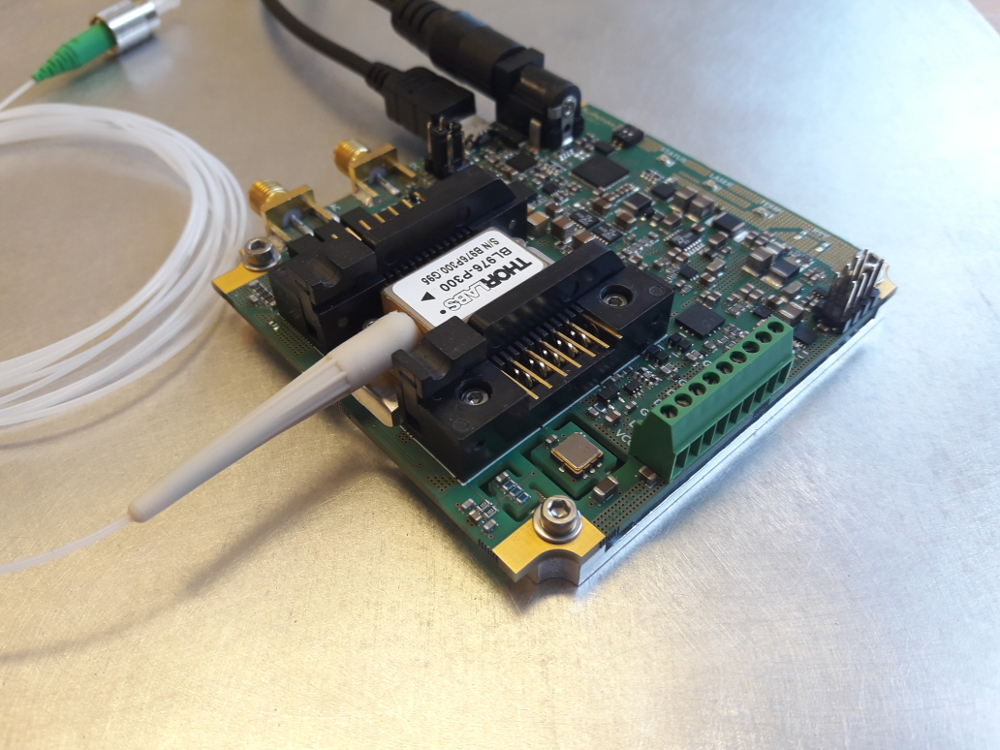### Super Luminescent Diode

The first graph shows the I-V characteristic of a Thorlabs SLD830S-A20 830 nm Super Luminescent Diode (SLED). As expected, the curve is very smooth since the diode only exhibits amplified spontaneous emission. We can see the laser voltage decreases when the temperature increases.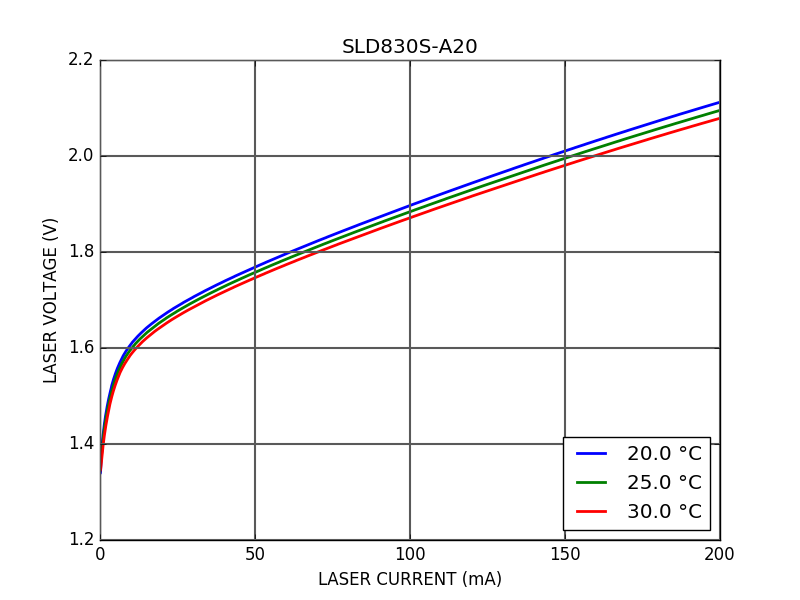### External Cavity Single Frequency Laser

The second diode is a Thorlabs SFL1550P external cavity single frequency laser operating at 1550 nm. The external cavity gives this laser a very good spectral purity (linewidth smaller than 100 kHz). This laser has a smaller voltage than the SLED. This can be explained by the higher wavelength ($eV = hc/\lambda$).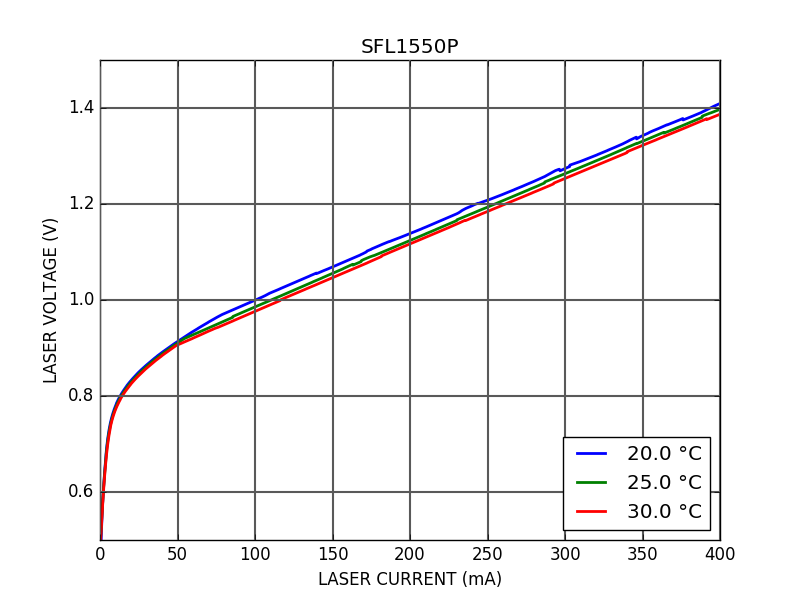On the zoomed curve below, we can observe that the laser voltage suddenly jumps when increasing the laser current. When the current increases, the wavelength drifts until the lasing mode is no longer the mode with the highest gain. At this moment, the laser transfers quickly its power to the higher gain mode. This causes a shift in optical power that can be observed on the laser voltage. This phenomenon is called mode hopping.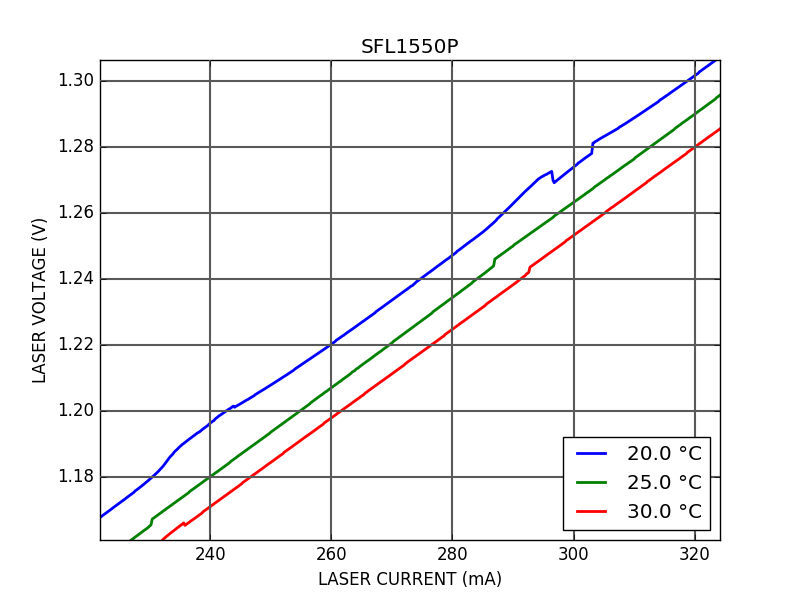### FBG-Stabilized Laser Diode

The third diode is a Thorlabs BL976-P300 Fiber Bragg Grating (FBG) Stabilized Laser Diode. It operates at 976 nm and can output up to 300 mW, which makes it a good pump diode for Erbium Doped Fiber Amplifiers (EDFAs).

The I-V characteristic curve has two well defined zones separated by the lasing threshold around 40 mA. Below the lasing threshold, it seems to behave similarly to the two previous diodes. At the threshold, the product $\lambda V = 1.31 \times 0.976 = 1.28$ µm V is close to $hc/e = 1.24$ µm V. Above the threshold, the behavior is linear with a slope of 0.625 V / A. This dynamic resistor of 0.625 Ω is related to thermal losses. It is two times smaller than the External Cavity Laser's thermal losses. This can be explained by the fact that the External Cavity Laser was optimized for spectral purity over thermal efficiency.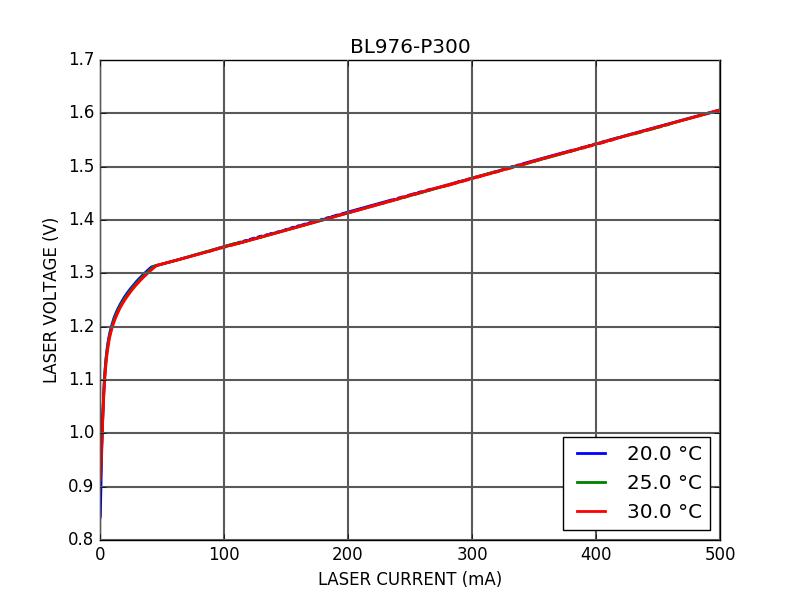On the zoomed curve below, we can see that once the laser threshold is crossed, the laser voltage no longer depends on the temperature. Compared to the External Cavity Laser, mode hops seem to be more frequent but disappear in the operating region above 400 mA.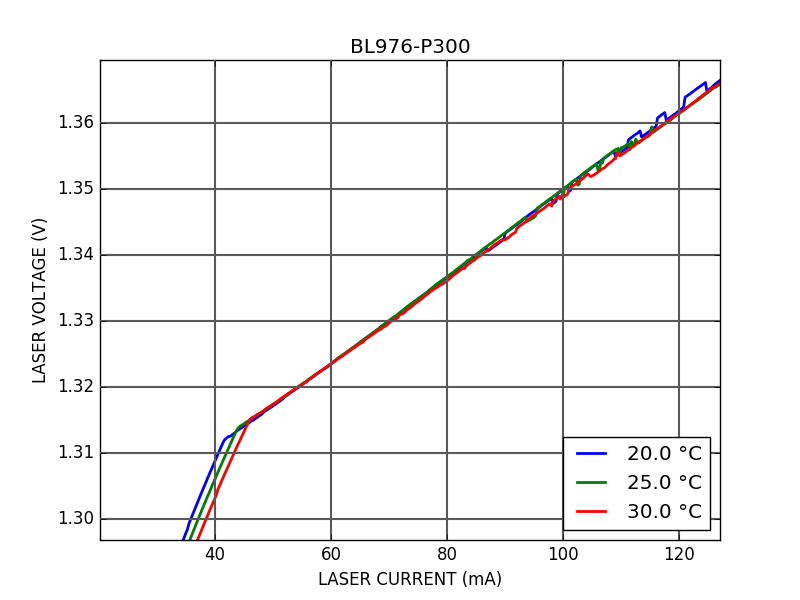## Python code

import time
import numpy as np
from serial import Serial

class CTL200(object):
def __init__(self, port='COM1'):
self.ser = Serial(port, 115200, timeout=1)

def send(self, _str):
self.ser.write(b"%s\r\n" % _str.encode())
return line.decode()

driver = CTL200(port='/dev/ttyUSB0')

tsets = np.array([20.0, 25.0, 30.0])
ilasers = np.arange(2000) * 0.25 + 0.0
vlasers = np.zeros((np.size(ilasers),np.size(tsets)))

driver.send("dgain %f" % 0)
driver.send("ilaser %f" % 0)
driver.send("lason %u" % 1)

for j, tset in enumerate(tsets):
driver.send("ilaser %f" % ilasers)
driver.send("tset %f" % tset)
time.sleep(30)
for i, ilaser in enumerate(ilasers):
driver.send("ilaser %f" % ilaser)
time.sleep(0.1)
vlaser = float(driver.send("vlaser"))
iphd = float(driver.send("iphd"))
print ilaser, vlaser, iphd, float(driver.send("rtact"))
vlasers[i,j] = vlaser
iphds[i,j] = iphd

driver.send("ilaser %f" % 0)

np.save('iv_curve.npy', (tsets, ilasers, vlasers))